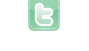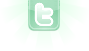## Weird rotation problem.

I have so when the user left clicks it (is supposed to) shoot a red ball directed at the mouse. This is the code for the ball actor.
```import greenfoot.*;  // (World, Actor, GreenfootImage, Greenfoot and MouseInfo)

/**
* Write a description of class redportal_ball here.
*
* @version (a version number or a date)
*/
public class redportal_ball extends Actor
{
/**
* Act - do whatever the redportal_ball wants to do. This method is called whenever
* the 'Act' or 'Run' button gets pressed in the environment.
*/

public MouseInfo mouse = Greenfoot.getMouseInfo();
public GameWorld board = (GameWorld) getWorld();
public boolean created = false;

public void act()
{
move(1);
Actor wall;
//wall = getOneObjectAtOffset(0, 0, wall.class);
if (getOneObjectAtOffset(0, 0, wall.class) != null)
{
board.removeObjects(board.getObjects(redportal.class));
redportal portal = new redportal();
board.removeObject(this);
}
if (!created)
{
setRotation(-45);
}

created = true;
}

public int findangle()
{
int mouseX = mouse.getX();
int mouseY = mouse.getY();
double deltaX = (mouseX  - this.getX());
double deltaY = (mouseY - this.getY());
double angle = Math.atan(deltaX/deltaY);
angle = angle * 180 / 3.14;
int angle_return = (int)(angle);
System.out.println(angle_return + " " + angle + " " + mouseX + " " + mouseY);
return angle_return;
}
}```
It only seems to shoot at either 90, 45, or 0 degree angles. Any reason?
```    public int findangle()
{
mouse = Greenfoot.getMouseInfo();
[...]
}
```
You need to refresh your MouseInfo otherwise you only turn to the position the mouse had when the scenario started. You also need to check that the mouse changed since your last refresh (i.e. with Greenfoot.mouseMoved(null)) otherwise Greenfoot.getMouseInfo() returns null and you'll get a null pointer.
You're only moving one cell (line 22). If you only move one cell, it's not possible to move any direction other than a multiple of 45 degrees. Imagine: ( ) ( ) ( ) ( ) (X) ( ) ( ) ( ) ( ) The 'X' can only move 0, 45, 90, ... 315 degrees. Two solutions: 1. move by a larger amount 2. import and use the SmoothMover class (Greenfoot 2.2.0, or copy it from another scenario)
Thanks davmac! That (move more) solution worked! It can now move at angles other than 0,45,90, etc, but it doesn't move exactly where I click (supposed to shoot a ball from the player to the mouse). This is the code I am using to find the angle between the mouse location and itself (which is created at the player's coordinates).
```    public int findangle()
{
int mouseX = mouse.getX();
int mouseY = mouse.getY();
double deltaX = (mouseX  - this.getX());
double deltaY = (mouseY - this.getY());
double angle = Math.atan(deltaX/deltaY);
angle = angle * 180 / 3.14;
int angle_return = (int)(angle);
System.out.println(angle_return + " " + angle + " " + mouseX + " " + mouseY);
return angle_return;
}```
There are a few possibilities. For one, you should probably use Math.atan2(...) to calculate the angle. See The Sinepost entry. Also, you should use Math.toDegrees(...) to convert the angle from radians to degrees, rather than just multiplying by 180 and dividing by 3.14 (by the way, there's also a 'Math.PI' constant...) Finally, as the angle returned is an int, it can only be whole numbers of degrees - 0, 1, 2 ... etc. It might be that this isn't precise enough for the accuracy you want.
Any way I could increase the accuracy? Int is the only accepted data type for setRotation(), so it would seem to be a roadblock. Any ideas? New Code:
```    public int findangle()
{
int mouseX = mouse.getX();
int mouseY = mouse.getY();
double deltaX = (mouseX  - this.getX());    //Change in X
double deltaY = (mouseY - this.getY());     //Change in Y
double angle = Math.atan2(deltaY, deltaX);    //Finds angle with deltaX and deltaY
angle = Math.toDegrees(angle);
int angle_return = (int)(angle);
System.out.println(angle_return + " " + angle + " " + mouseX + " " + mouseY);
return angle_return;
}```
Store the rotation as a double, and calculate the movement amounts yourself (and use setLocation()) rather than using move().